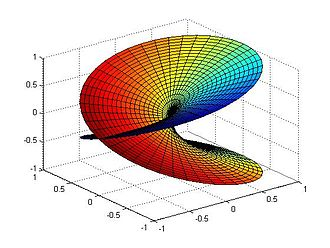Riemann surface for the function $f(z)=\sqrt{z}.$ The two horizontal axes represent the real and imaginary parts of z, while the vertical axis represents the real part of $\sqrt{z}.$ For the imaginary part of $\sqrt{z},$ rotate the plot 180° around the vertical axis.
A Riemann surface (after Bernhard Riemann) is a one-dimensional complex manifold. Riemann surfaces can be thought of as deformed versions of the complex plane: locally near every point they look like patches of the complex plane, but the global topology can be quite different. For example, they can look like a sphere or a torus or several sheets glued together.

The main point of Riemann surfaces is that holomorphic functions may be defined between them. Riemann surfaces are nowadays considered the natural setting for studying the global behavior of these functions, especially multi-valued functions such as the square root and other algebraic functions, or the logarithm.

Every Riemann surface is a two-dimensional real analytic manifold (i.e., a surface), but it contains more structure (specifically a complex structure) which is needed for the unambiguous definition of holomorphic functions. A two-dimensional real manifold can be turned into a Riemann surface (usually in several inequivalent ways) if and only if it is orientable and metrizable. So the sphere and torus admit complex structures, but the Möbius strip, Klein bottle and projective plane do not.

AGentleIntroductionToTimeComplexity
AbsoluteValue
AlgebraicNumber
BirthdayProblem
EuclideanAlgorithm
EulersNumber
ImaginaryNumber
IrrationalNumber
MagnitudeOfAVector
MathematicsTaxonomy
NaturalLogarithm
RiemannHypothesis
Root
RootTwoIsIrrational
Surd
(none) (none)
Logarithm
SquareRoot
InverseFunction

## You are here

RiemannSurface
BernhardRiemann
ComplexPlane
Function
HolomorphicFunction
InverseFunction
PolarRepresentationOfAComplexNumber
Root
CoDomainOfAFunction
ComplexNumber
DomainOfAFunction
ImageOfAFunction
ImaginaryNumber
RealNumber
ArgandDiagram
Euclid
Polynomial
RangeOfAFunction